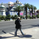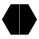3795 views
3795
Auto SR based on fractals and volume .

http://synapticex.com
//@version=2
//synapticex.com
study("Fractal Support Resistance", shorttitle="FSR", overlay=true)
tf = input(title="Resolution", type=resolution, defval = "current")
vamp = input(title="VolumeMA", type=integer, defval=6)
vam = sma(volume, vamp)

up = high>high and high>high and high<high and high<high and volume>vam
down = low<low and low<low and low>low and low>low and volume>vam
fractalup =  up ? high : fractalup
fractaldown = down ? low : fractaldown

fuptf = security(tickerid,tf == "current" ? period : tf, fractalup)
fdowntf = security(tickerid,tf == "current" ? period : tf, fractaldown)

plot(fuptf, "FractalUp", color=lime, linewidth=1, style=cross, transp=0, offset =-3, join=false)
plot(fdowntf, "FractalDown", color=red, linewidth=1, style=cross, transp=0, offset=-3, join=false)Hey @synapticEx great indi, been using it for a while now! Can I ask, is it possible to make an update to the indicator so that it does not affect the scaling of the chart? For example, if the indi is set up 4 hour timeframe S/R's.. and you're on say a <30m timeframe - the chart will squish up to fit the 4hour Resistance, for example. Hope this makes sense! Thanking you in advance